Test: Electrostatics - 1

# Test: Electrostatics - 1

Test Description

## 10 Questions MCQ Test Topicwise Question Bank for Electrical Engineering | Test: Electrostatics - 1

Test: Electrostatics - 1 for Electrical Engineering (EE) 2023 is part of Topicwise Question Bank for Electrical Engineering preparation. The Test: Electrostatics - 1 questions and answers have been prepared according to the Electrical Engineering (EE) exam syllabus.The Test: Electrostatics - 1 MCQs are made for Electrical Engineering (EE) 2023 Exam. Find important definitions, questions, notes, meanings, examples, exercises, MCQs and online tests for Test: Electrostatics - 1 below.
Solutions of Test: Electrostatics - 1 questions in English are available as part of our Topicwise Question Bank for Electrical Engineering for Electrical Engineering (EE) & Test: Electrostatics - 1 solutions in Hindi for Topicwise Question Bank for Electrical Engineering course. Download more important topics, notes, lectures and mock test series for Electrical Engineering (EE) Exam by signing up for free. Attempt Test: Electrostatics - 1 | 10 questions in 30 minutes | Mock test for Electrical Engineering (EE) preparation | Free important questions MCQ to study Topicwise Question Bank for Electrical Engineering for Electrical Engineering (EE) Exam | Download free PDF with solutions
 1 Crore+ students have signed up on EduRev. Have you?
Test: Electrostatics - 1 - Question 1

### What is the ratio of electrostatic repulsion and gravitational attraction between two electrons? Given: Q = Charge on an electron = 1.6 x 10-19C G = Gravitation constant = 6.67 x 10-11 Nm2/kg2 m = Mass of an electron = 9.1 x 10-31 kg

Detailed Solution for Test: Electrostatics - 1 - Question 1

By columb’s law,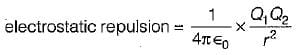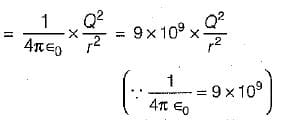Also, by Newton's law of gravitation,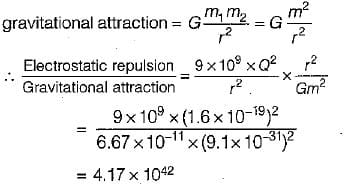Test: Electrostatics - 1 - Question 2

### What is the electric field strength at a distance of 200 mm from a charge of 2 x 10-6 Coulomb, in vacuum?

Detailed Solution for Test: Electrostatics - 1 - Question 2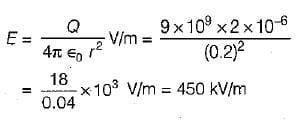Test: Electrostatics - 1 - Question 3

### Two point charges of 3 x 10-9 C and -2 x 10-9C are spaced two meters apart. What is the electric field at a point which is one meter from each of the two point charges?

Detailed Solution for Test: Electrostatics - 1 - Question 3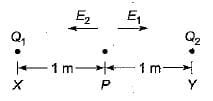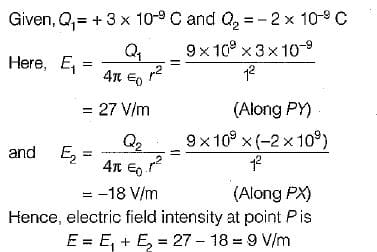Test: Electrostatics - 1 - Question 4

Five equal point charges of Q = 20 x 10-9C, are placed at x = 2,3,4,5 and 6 cm. The electric potential at origin will be

Detailed Solution for Test: Electrostatics - 1 - Question 4

Electric potential at origin is given as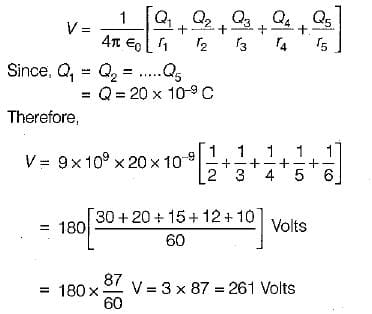Test: Electrostatics - 1 - Question 5

What is the equivalent capacitance of a parallel plate capacitor having two dielectrics, ∈r1 = 1.5 and ∈r2 = 3.5, each comprising one-half the volume as shown in figure below with A = 2 m2 and d = 1 mm?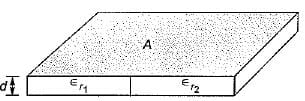Detailed Solution for Test: Electrostatics - 1 - Question 5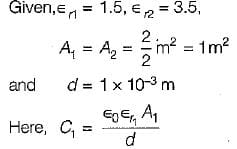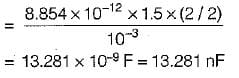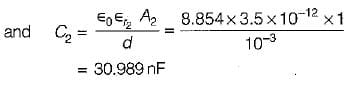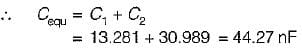Test: Electrostatics - 1 - Question 6

Four point charges 1, - 2, -3 and 4 μC are located on the x-axis at x = 1, 2, 3 and 4 metre respectively. The energy stored in the field is

Detailed Solution for Test: Electrostatics - 1 - Question 6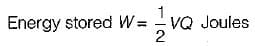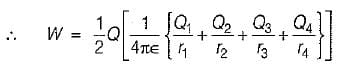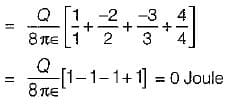Test: Electrostatics - 1 - Question 7

The electric field strength at a point in front of an infinite sheet of charge is

Detailed Solution for Test: Electrostatics - 1 - Question 7

The electric field strength at a point in front of an infinite sheet of charge is given by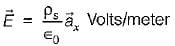where, ρs = charge density and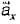= unit vector normal to the sheet and directed away from the sheet.
Here,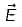is independent of the distance of the point from the sheet.

Test: Electrostatics - 1 - Question 8

Assertion (A): If all the points in space which have the same potential are jointed, then equipotential surfaces are obtained.
Reason (R): The field and the equipotential surfaces are orthogonal to each other.

Detailed Solution for Test: Electrostatics - 1 - Question 8

Both assertion and reason are individually true. However, the correct reason for assertion is that equipotential surfaces are those on which the potential is everywhere the same. Thus, if all the points in space which have the same potential are joined, then equipotential surfaces are obtained.

Test: Electrostatics - 1 - Question 9

Consider the following statements associated with various medias encountered in electostatics:
1. An isotropic media is one whose properties are independent of direction
2. A homogeneous media is one whose physical characteristics vary from point-to- point.
3. A non-homogenous media is one whose physical characteristics do not vary from point-to-point:
4. A linear media is a one in which the electric flux density is proportional to the electric field intensity.

Which of the above statements are correct?

Detailed Solution for Test: Electrostatics - 1 - Question 9

Statements 2 and 3 are not correct because a homogenous media is one whose physical characteristics do not vary from point-to-point while a non-homogeneous media is one whose physical characteristics vary from point-to-point.

Test: Electrostatics - 1 - Question 10

Assertion (A): Continuity of current equation is derived from law of conservation of charge.
Reason (R): Continuity of current equation states that there can be no accumulation of charge at any point.

## Topicwise Question Bank for Electrical Engineering

207 tests
 Use Code STAYHOME200 and get INR 200 additional OFF Use Coupon Code
Information about Test: Electrostatics - 1 Page
In this test you can find the Exam questions for Test: Electrostatics - 1 solved & explained in the simplest way possible. Besides giving Questions and answers for Test: Electrostatics - 1, EduRev gives you an ample number of Online tests for practice

207 tests